Example

####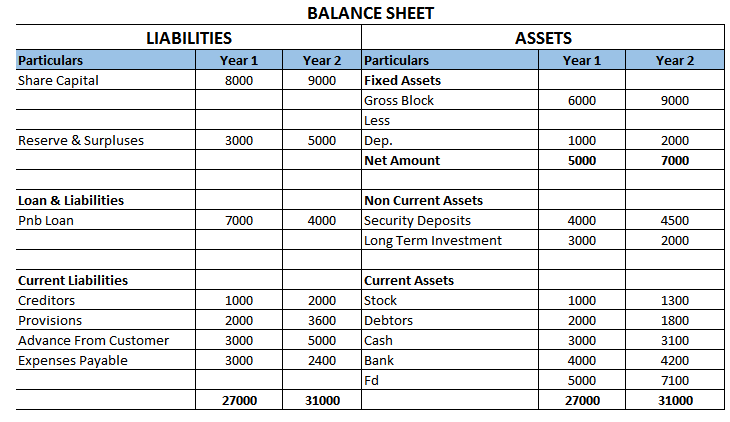(adsbygoogle = window.adsbygoogle || []).push({});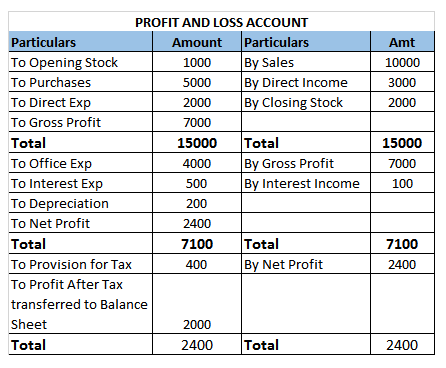#### Step 2 Calculate Sources and Uses of Cash

In Fund Flow, there were 4 heads

1. Long Term Sources
2. Long Term Uses
3. Short Term Sources
4. Short Term Uses

However in Cash Fow,there are only 2 heads

1. Sources of Cash
2. Uses of Cash

Hence, both Long Term and Short Term Sources are called Sources of Cash

Similarly, both Long Term and Short Term Uses are called Uses of Cash

Rules are Same as Fund Flow

How to Determine Sources and Uses of Cash?

 Case m Source/ Use If Liability Increase Source If Liability Decrease Use If Assets Increase Use If Assets Decrease Source

Check:- Ensure that Sources of Cash are equal to Uses of Cash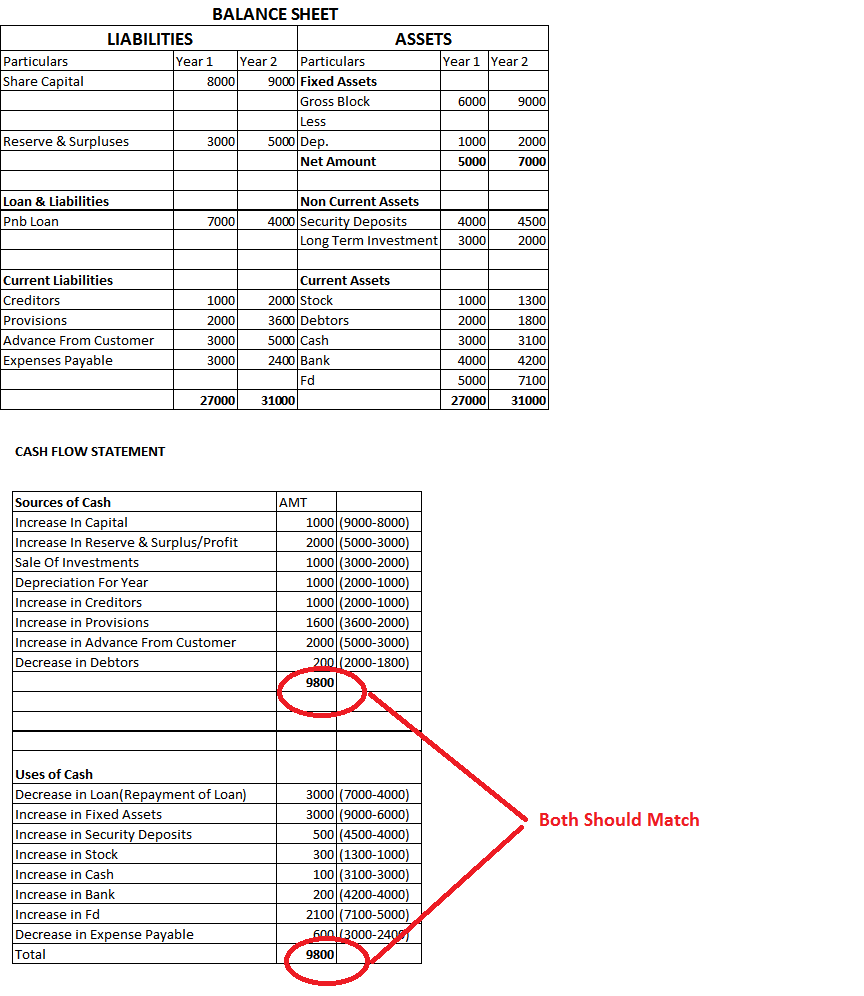#### Step 3  Instead of Increase in Current Assets, Calculate Increase in Current Assets except Cash and Cash Equivalents

(Except Cash, Bank, FD)

Now Sources and Uses will not match

Also Calculate/Deficit Surplus of Cash (Sources-Uses of Cash)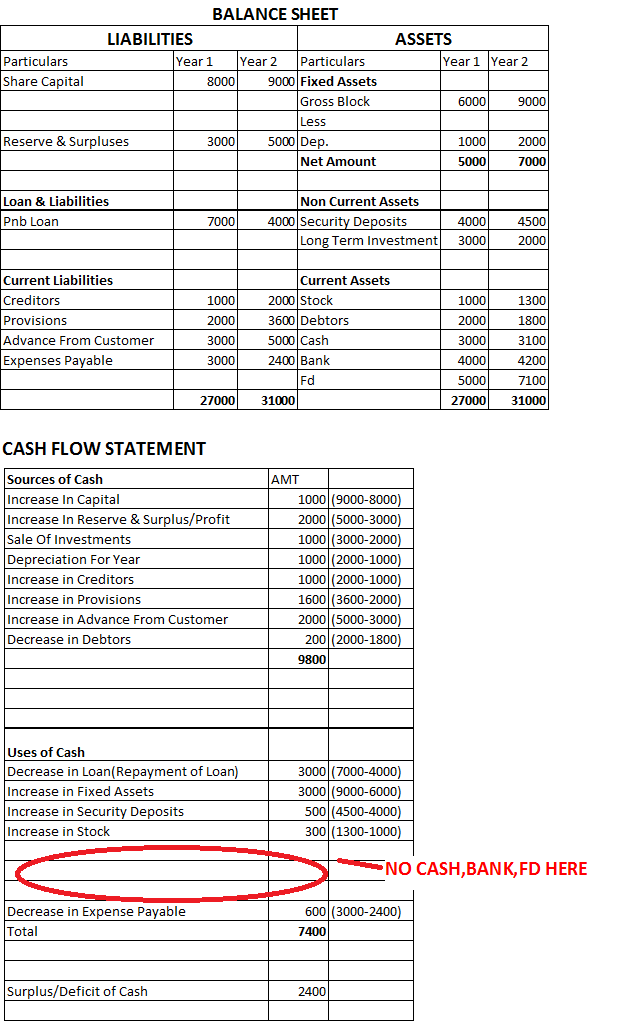#### Step 4 Add Opening Balance Of Cash and Cash Equivalents to Surplus to get Closing Balance of Cash

If it is matching with Current year Cash,Bank,FD as shown in Balance Sheet, it means Cash Flow is Correctly prepared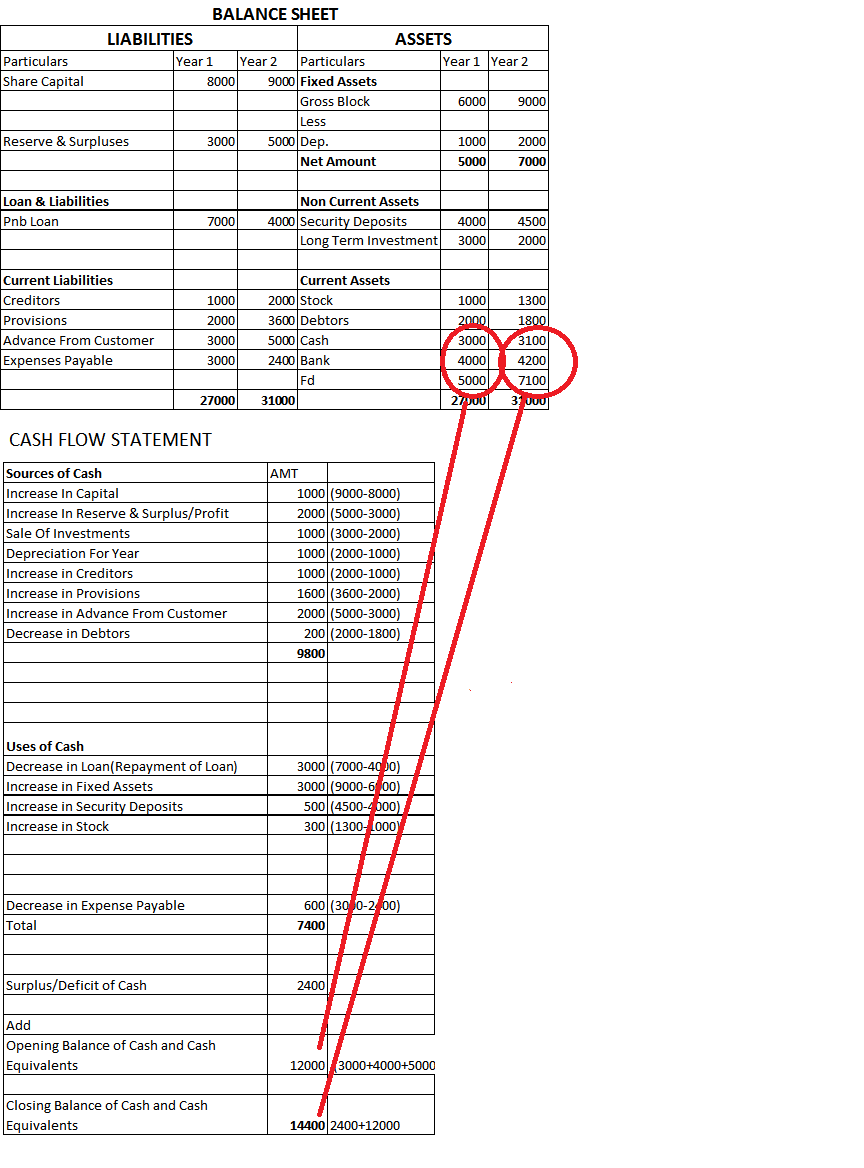#### Step 5 Note Following figures from Profit and Loss

• Profit After Tax
• Interest Expense
• Interest Income
• Tax Expense

And Calculate Operating Profit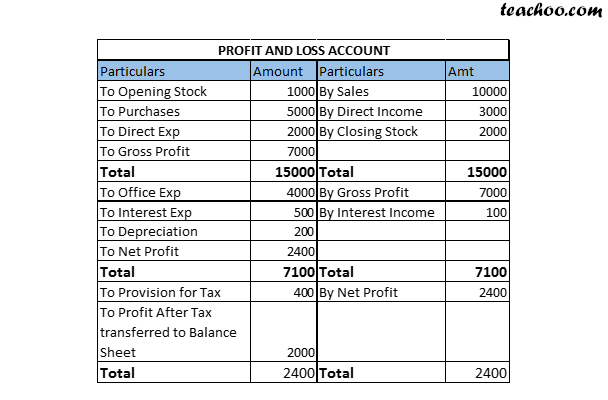In our case

• Profit After Tax=2000
• Interest Expense=500
• Interest Income=100
• Tax Expense=400

Operating Profit=Profit After Tax + Tax Expense + Interest Expense –Interest Income

=2000+400+500-100

=2800

#### Step 6 Replace Profit as Appearing in Cash Flow Statement with Operating Profit.

Also Show Interest Income as Source and Interest Expense and Tax as Use

There is no Net Effect of this in Cash Flow:-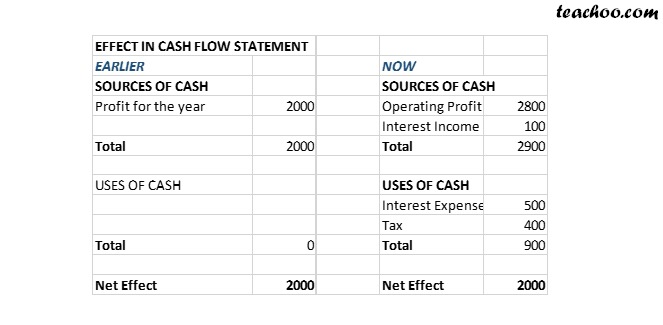Cash Flow Statement will appear like this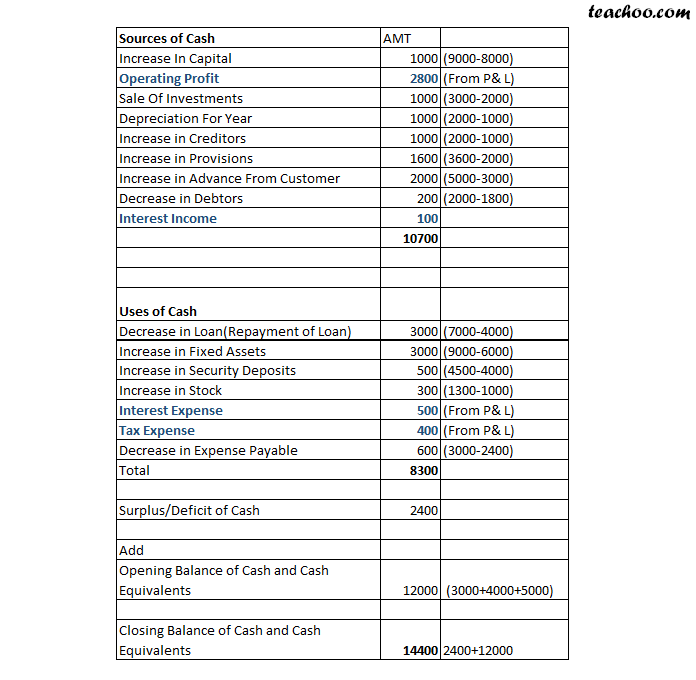This is our Final Cash Flow Statement

1. Accounts and Finance
2. Step 6 Fund Flow and Cash Flow
3. Cash Flow Statement

Cash Flow Statement

Accounts and Finance
Step 6 Fund Flow and Cash Flow